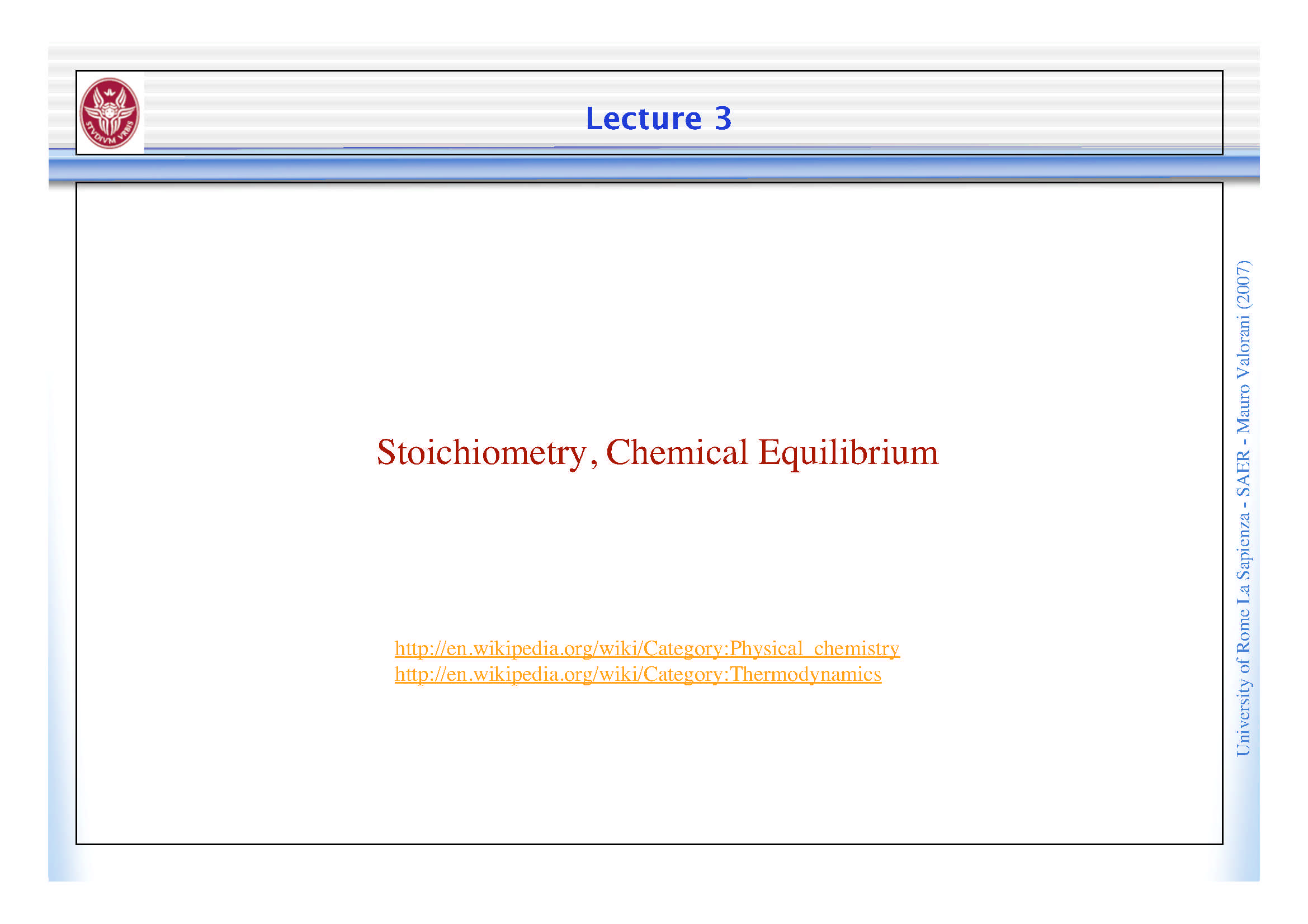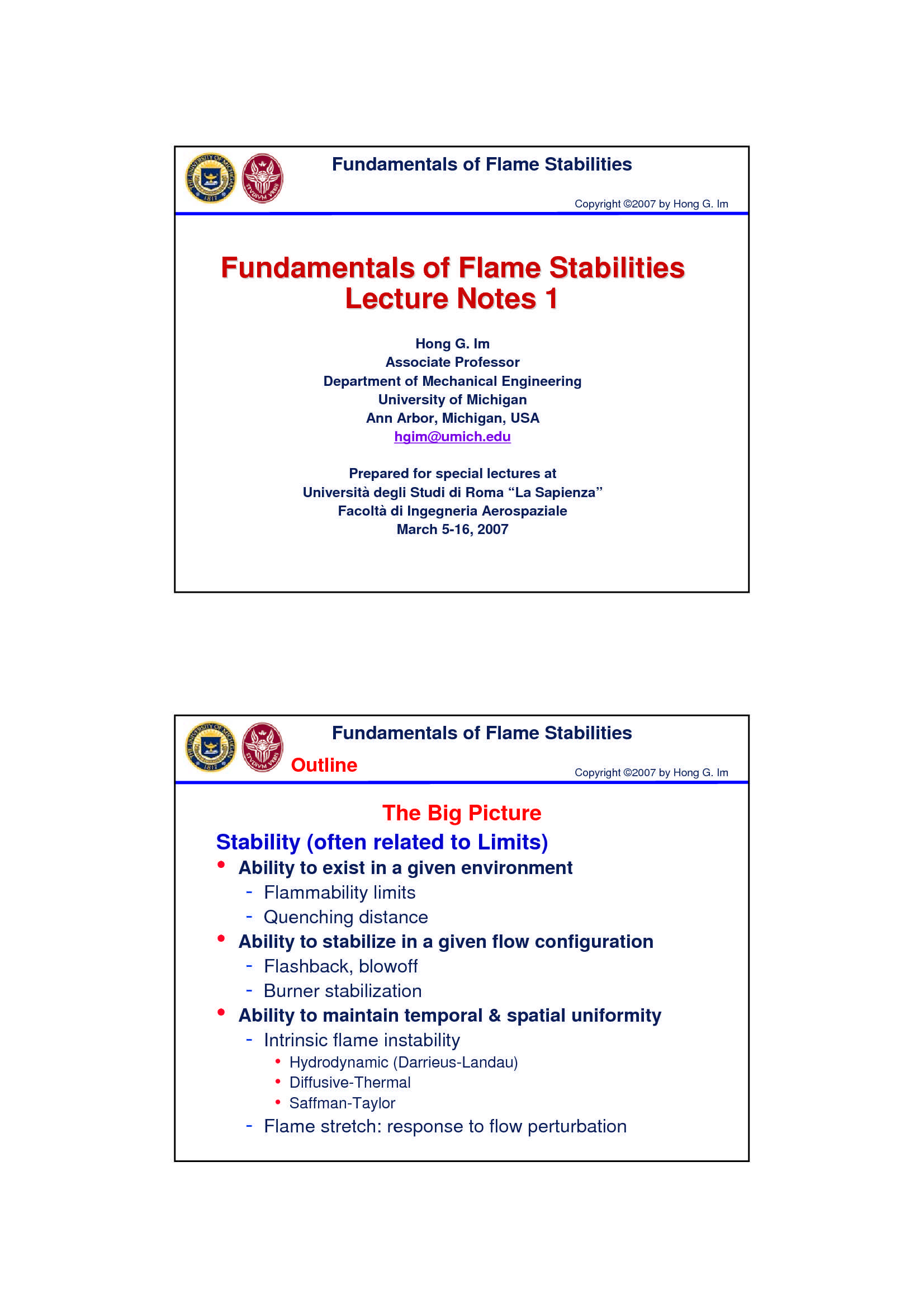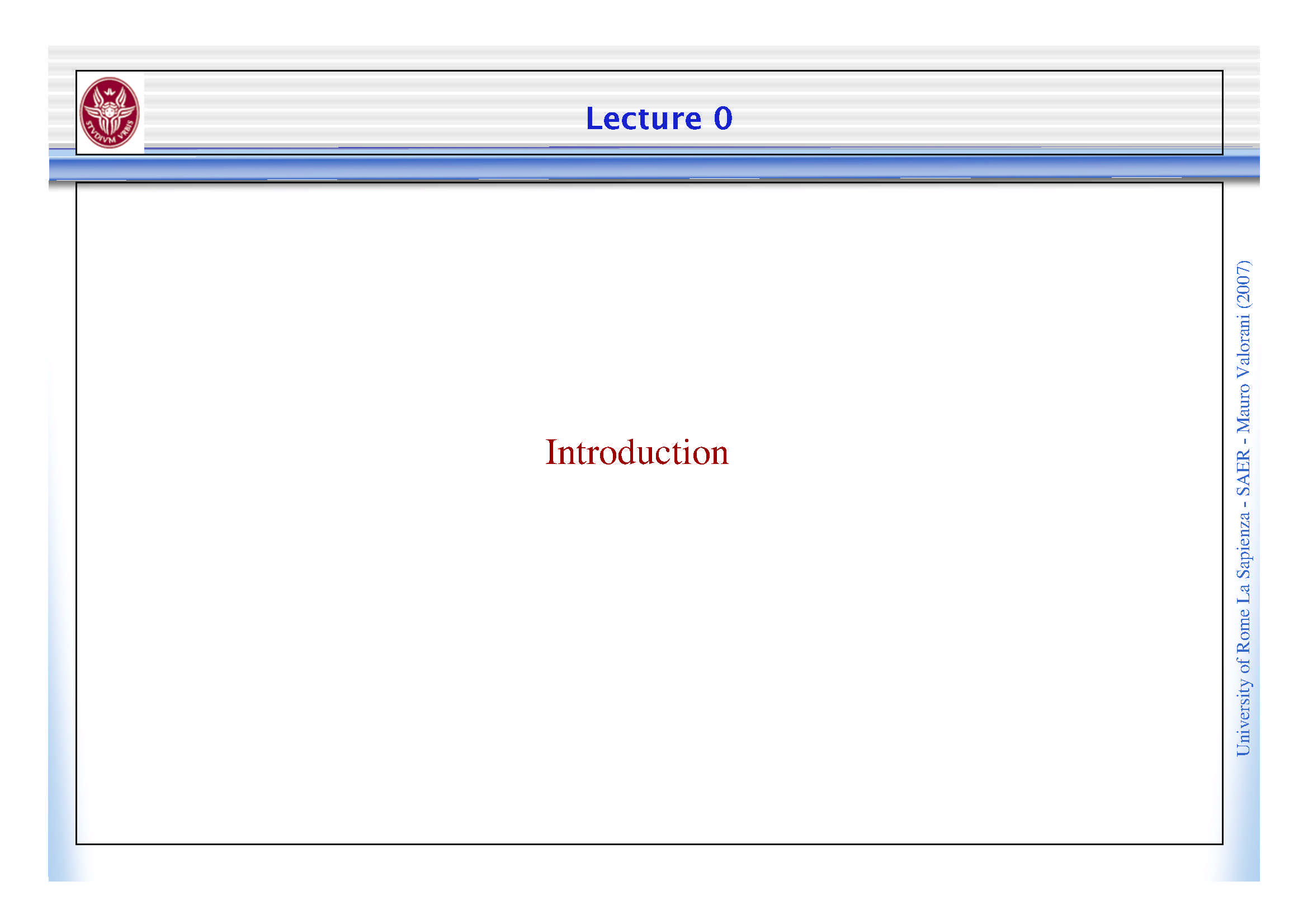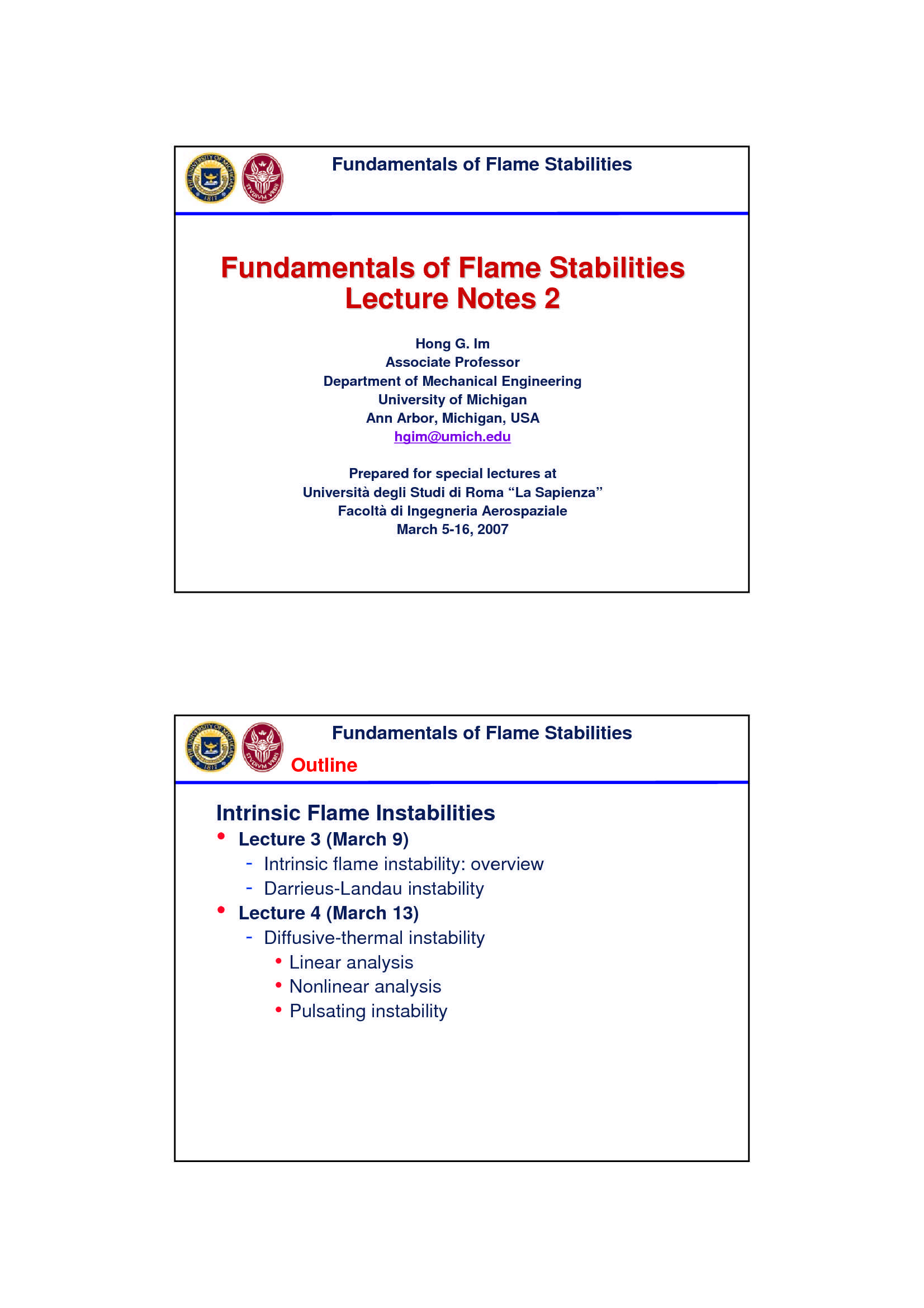Che materia stai cercando?

# Cinetica chimica

Materiale didattico per il corso di Combustione del Prof. Mauro Valorani, all'interno del quale sono affrontati i seguenti argomenti: cinetica chimica; processi irreversibili e reazioni chimiche; costanti di reazione; classificazione delle reazioni; reazioni lente e reazioni rapide.

Esame di Combustione docente Prof. M. Valorani

Anteprima

### ESTRATTO DOCUMENTO

Why we need Chemical Kinetics

Enforcing atomic mass conservation yields the rate equations describing the time evolution of the

composition: (2007)

" k

dN d

!

= Valorani

## S

k

dt dt

k Mauro

ξ k

The time rate of change of the progress variable needs to be related to the state of the system -

(p,T,N ), according to a constitutive law of the form: SAER

j -

! Sapienza

k

d = = "

k fk bk

r ( p,T , N ) r ( p,T , N ) r ( p,T , N )

j j j

dt La

Rome

Aim of chemical kinetics is to find explicit forms of the constitutive laws for the forward and of

backward(reverse) rates of a reaction University

Irreversible Processes: Chemical Reactions

The symbolic formula describing a reaction between m moles of reactant A and n moles of

reactanct B, yieldings p moles of product C and q moles of reactant D, is written as: (2007)

!

!!

"

Kf

+ +

#

!!

! Valorani

m A n B p C q D

Kb

where:

1) K , K indicate the reaction constants of the forward (left arrow) and backward (reverse, Mauro

f b

right arrow) reactions, respectively; -

2) The stoichiometric coefficients are conventionally assumed: m, n < 0 ; p, q > 0. SAER

The main result of chemical kinetics is the explicit form of the reaction rates: -

Sapienza

= =

m n p q

## r K (T )[A] [B] r K (T )[C] [D]

f f b b La

p p X Rome

= = " =

j j

from: [X ] r r(T , p, X )

## !T !T

j j of

University

The exponents n and m are called reaction orders and depend on the reaction mechanism. The

stoichiometric coefficients and reaction orders are very often equal, but only in one step reactions,

molecularity (number of molecules or atoms actually colliding), stoichiometry and reaction order

must be the same. Molecularity

Molecularity chemistry molecular entities

in is the number of colliding that are

• reaction step

involved in a single (2007)

Valorani

the molecularity is a theoretical concept and can only be applied to elementary

• reactions Mauro

In elementary reactions, the reaction order, the molecularity and the stoichiometric

• -

## SAER

coefficient are the same, although only numerically, because they are different

concepts -

Sapienza

 A reaction involving one molecular entity is called unimolecular. La

Rome

 A reaction involving two molecular entities is called bimolecular.

 of

A reaction involving three molecular entities is called termolecular. University

Reaction Order

Order of reaction

The with respect to a certain reactant is defined, in chemical

• kinetics, as the power to which its concentration term in the rate equation is raised: (2007)

dc ! n

i c n reaction order wrt to species j Valorani

j

dt → 1 2

For example, given a chemical reaction A + 2B C with a rate equation r = k[A] [B] ,

• Mauro

order with respect to

the reaction A would be 1 and with respect to B would be 2,

total reaction order -

the would be 2+1=3. SAER

n

" %

dc ! -

Sapienza

!

i c n total reaction order

A A B B & )

m m Valorani

## A B

A steric factor Mauro

! !

, diameters of molecules of type A and B

## A B

m , m mass of molecules of type A and B -

## A B

E activation energy

a -

Sapienza

Empirical formulas (Arrhenius type):

• La

E Rome

" a

!

= #T

K (T ) A T e of

A steric factor University

E activation energy

a

! temperature dependence

Activation Energy, Transition State & Enthalpy of Combustion

activation energy

The is the threshold energy, or the energy that must be overcome

• in order for a chemical reaction to occur. Activation energy may otherwise be (2007)

denoted as the minimum energy necessary for a specific chemical reaction to occur. Valorani

The transition state along a reaction coordinate is the point of maximum free

• energy, where bond-making and bond-breaking are balanced. Mauro

-

## SAER

-

Sapienza

La

Rome

of

University

Rates of Change of Species

For any reaction of the form: !

!!

"

Kf

+ +

#

!!

!

m A n B p C q D (2007)

Kb

the stoichiometric constraint yields: Valorani

" { }

1 d[A] 1 d[B] 1 d[C] 1 d[D] d Mauro

! = ! =+ =+ = = !

r (T ,[A],[B]) r (T ,[C],[D])

f b

m dt n dt p dt q dt dt -

## SAER

enabling to find the rate of change of each species involved in that reaction: -

Sapienza

( )

d[A] = !m ! = !m !

m n p q

(r r ) K (T )[A] [B] K (T )[C] [D]

f b f b

dt La

( ) Rome

d[B] = !n ! = !n !

m n p q

(r r ) K (T )[A] [B] K (T )[C] [D]

f b f b

dt of

University

( )

d[C] = ! = !

m n p q

p (r r ) p K (T )[A] [B] K (T )[C] [D]

f b f b

dt ( )

d[D] = ! = !

m n p q

q (r r ) q K (T )[A] [B] K (T )[C] [D]

f b f b

dt

Equilibrium Condition as Asymptotic Solution of Kinetics

" ( )

k

dN d

! ! !

= = = #

k fk bk

S S r ( p,T , n ) S r r (2007)

k k j k

dt dt

k k k Valorani

j

dN = & # '

fk bk Mauro

lim 0 r r 0

( ) & )

%G Mauro

b #

" k ok

## !T

K (T ) \$

(T ) ( )

K (T ) j #

" k

" !T

c ' *

Exp j

p j !T j -

( + SAER

-

Sapienza

NB: La

K is a kinetic information, Rome

f

K is a thermochemical information

p of

University

PAGINE

23

PESO

2.07 MB

AUTORE

PUBBLICATO

+1 anno fa

DETTAGLI
Esame: Combustione
Corso di laurea: Corso di laurea magistrale in ingegneria aeronautica
SSD:
A.A.: 2007-2008

I contenuti di questa pagina costituiscono rielaborazioni personali del Publisher Atreyu di informazioni apprese con la frequenza delle lezioni di Combustione e studio autonomo di eventuali libri di riferimento in preparazione dell'esame finale o della tesi. Non devono intendersi come materiale ufficiale dell'università La Sapienza - Uniroma1 o del prof Valorani Mauro.

Acquista con carta o conto PayPal

Scarica il file tutte le volte che vuoi

Paga con un conto PayPal per usufruire della garanzia Soddisfatto o rimborsato

RecensioniTi è piaciuto questo appunto? Valutalo!

## Altri appunti di CombustioneDispensa

### Stechiometria ed equilibrio chimicoDispensa

### Stabilità della fiammaDispensa

### Combustione omogenea e combustione eterogeneaDispensa